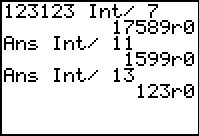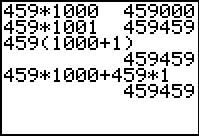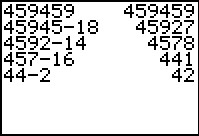# Activities

••• ##### Subject Area

• Math: Middle Grades Math: Number Sense

• ##### Author6-8

45 Minutes

• ##### Device
• TI-73 Explorer™
• TI-Navigator™
• ##### Software

LearningCheck™ Creator

## A Prime Investigation with 7, 11, and 13#### Activity Overview

In this activity, students will investigate the divisibility of 7, 11, and 13 and discover the divisibility characteristics of certain six-digit numbers. They will use the Integer Division feature of the TI-73 Explorer™.

#### Key Steps

•Students begin by using the Integer Division feature to explore the divisibility of a 3-digit number that is repeated to form a six-digit number by 7, 11, and 13. The Integer Division feature displays the quotient and the remainder. If there is no remainder, the calculator will display a zero.

•With the distributive property, students will discover why a 3-digit number repeated is always divisible by the product of 7, 11, and 13.

•Then students explore various divisibility rules, such as when numbers are divisible by 2, 3, 5, 7, and 11. Finally students develop their own divisibility rule.